Here’s the theory of relativity in 500 words – Kevin Drum Skip to content

# Here’s the theory of relativity in 500 words

Bob Somerby keeps asking for an understandable explanation of the theory of relativity. In this post, I shall try to oblige him in less than 500 words. Note, however, that I am taking an, um, nontraditional approach.

Special Relativity

1. Until 1905, everyone thought that velocities added up. That is, if you're walking at 2 mph and you get on an escalator that's moving 2 mph, your total velocity is 4 mph. Simple.
2. Simple but wrong. In fact, your total velocity is a little less than 4 mph. It turns out that our intuitive sense of how the universe works is incorrect. Here is the actual formula for adding up velocities, where c is the speed of light: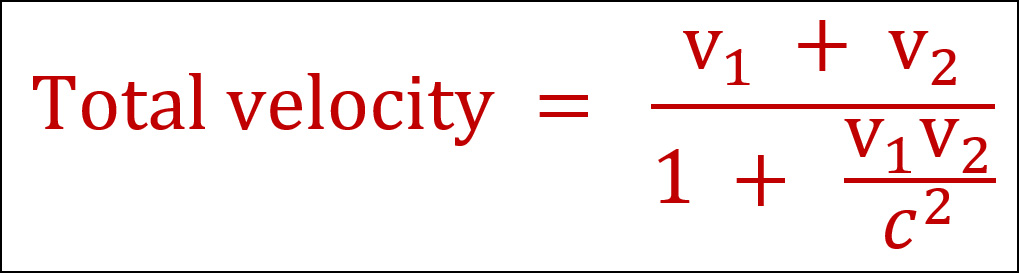3. Once you have this, the rest is just arithmetic. If you take this formula and apply it to objects in motion, all the weird predictions of relativity pop out: lengths get shorter as velocities increase; time slows down; nothing can go faster than the speed of light; and e=mc².

General Relativity (Gravity)

1. Until 1916, everyone thought that normal Euclidean geometry described how the universe worked.
2. It doesn't. Once again, our intuitive sense of how the universe works was wrong.
3. In fact, in the presence of mass (the earth, for example), geometry becomes non-Euclidean, or slightly "warped." Here is the Einstein field equation that describes the shape of space and time in the presence of mass: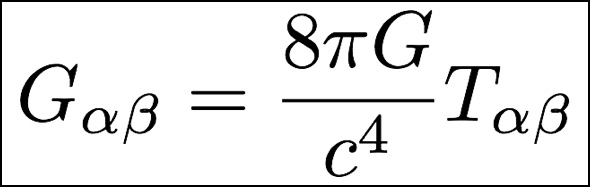4. This equation is even more complicated than it looks, but it basically says the curvature of spacetime (the curvature tensor Gαβ) equals the distribution of mass/energy (the metric tensor Tαβ).
5. The rest, as Einstein himself said, is just (very advanced) arithmetic. When you work through the consequences of warped spacetime, it turns out that time slows down in the presence of mass. Another way of saying this is that motion through time decreases.
6. Another consequence is that objects accelerate toward the mass. In other words, motion through space increases.
7. This is gravity: in the presence of mass, motion through time decreases (slightly) and is converted into motion through space.

You will notice that both cases have two things in common. First, the rules of how the universe works turn out to be a little more complicated than we thought. The old rules of Isaac Newton are almost right, but not quite.

Second, once you have the rules the rest is just working through the math. This might seem a bit like cheating, but it's nothing new. An ordinary freshman physics textbook spends hundreds and hundreds of pages working through the consequences of a few rules set down by Isaac Newton. But if you discover new rules, then you have to work through the math all over again and you'll come up with different results. That's what college textbooks on special and general relativity do for hundreds and hundreds of pages.

You're disappointed, aren't you? You really want to know what it all means. Sorry. There's really no deep philosophy here. For reasons no one entirely understands, we live in a universe ruled by the iron fist of mathematics.

In other words, once you know the rules, it's all just math, baby.

## 62 thoughts on “Here’s the theory of relativity in 500 words”

1.One other important Special Relativity feature is the unification of space and time. That leads to some important differences between everyday concepts like simultaneity and the corresponding relativistic versions.

1.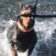Mathematically it is very elegant, as a point in space goes from being described as a three component vector (x, y, z) to a four component vetor (x,y, z, t). The relationship between momentum and kinetic energy is clarified, as kintetic energy becomes the fourth component of the momentum vector.

2.Not bad, but one minor point of correction - non-Euclidian geometries had been discovered and studied for several decades before 1916.

1.Well, while non-Euclidean geometry didn't really flourish until the 1800's, in fact it's centuries older than that. Arab mathematicians back in the 1400's and European mathematicians from the 1600's all explored the consequences of negating Euclid's fifth postulate. While the intent was typically to try to reach a contradiction and thereby prove the fifth postulate, that was, of course, never the result. They didn't know it, or call it that, but they were the pioneers of non-Euclidean geometry.

1.However, just correct it to "almost everyone" and it will be a pretty accurate description of where things were in 1916.

2.Non-Euclidian geometries had been discovered, but I don't believe anyone thought they were a useful model for real world space or space-time geometry.

1.Map-makers had been warping a grid onto a sphere for a hundred years at that point.

They just didn't think it was special in that the universe isn't square.

3.And David Hilbert (namesake of Kevin's cat) established the mathematical basis of quantum mechanics before the discovery of quantum mechanics.

3.Let me see if I understand something.

The denominator in that total velocity equation has a very narrow set of possible values, yes? If an object is stationary, v1 x v2 = 0, 0 / c^2 = 0, and 1 + 0 = 1.

On the other hand, if v1 x v2 is close to its maximum, it approaches c. c / c^2 = 1 / c, a tiny number. 1+ a / c is just barely above 1. So the boundaries of the denominator are within the interval [1, 1 + 1/c], yes?

1.edit: not 1 + a/c, 1 + 1/c

2.If either velocity is very small compared to c, then the denominator in the sum formula is approximately 1. This covers all day-to-day scenarios, and explains why the relativistic effect on velocity summation is normally negligible. It's only when both velocities are comparable to c that the denominator is significantly greater than 1. This covers the "What happens when you're in a car traveling near the speed of light and you turn on your headlights?" scenarios.

1.Oh! That calls attention to a small, significant detail. The numerator contains the sum of v1 and v2. The denominator contains the product of v1 and v2.

That makes a big difference.

If v1 and v2 are approaching c, the v1 + v2 approaches 2c. v1 x v2 approaches c^2. And c^2 / c^2 = 1. So we have 2c / 2 = c as the maximum velocity!

1.Now you've got it.

4."lengths get shorter as velocities increase". Perhaps another commenter can explain how this pops out of equation 1 using the same man walking on an escalator scenario.

1.That should be, "lengths appear to get shorter as velocities increase." While the decrease in length is "real" to the extent that an objective measurement would show it, from the point of view of the thing that is moving fast, there is no change. It's a matter of the frame of reference of the observer relative to that of the observed (hence, "relativity").

A slightly handwaving explanation gets into the space/time continuum stuff that I mentioned above:

You're traveling up a very long escalator. Mounted next to the escalator, and aligned with the escalator's direction of travel, is a very long ladder. As you move up the escalator, you see that you're approaching the ladder. Because the near end of the ladder is closer to you than the far end is, and because the speed of light is finite, light from the near end reaches your eyes before light from the far end does. So, during the interval between when the light from the near end of the ladder reaches your eyes and light from the far end of the ladder reaches your eyes, you've moved some distance up the escalator. In other words, by the time you see the far end, you're closer to it, and thus the ladder appears to be shorter than it is. The effect is, of course, negligible at ordinary speeds, but at speeds near the speed of light, other stuff happens as well: the ladder appears to curve and tilt more and more towards the escalator.

Again, the above contains some handwaving. You need to do the math to get a more correct answer.

1.I love this stuff. Isn't the real introduction to special relativity the point that the speed of light is constant?

Before being introduced to this world, a person tends to think that the speed of anything would be affected by the object that projects it - that somehow the light emanating from the headlights of a moving car would be the speed of light plus the speed of the car. When that turns out to be incorrect the fun begins, no?

1.Yes, the fact that the speed of light (in vacuum) is constant, no matter what, is the cornerstone of Special Relativity. Everything falls out from that, eventually.

2.but you still get there faster going faster 😉

3.Steve, thanks very much for taking the time.

4.Sorry, that's not correct. The effect you describe does happen, but that's separate from length contraction.

1.And Kevin's statement that the velocity addition formula implies the rest isn't really correct. The expression he gave is correct if you're walking in the direction of motion of the escalator, but it doesn't address what would happen if you walked at an angle with respect to the escalator direction (imagine a wide escalator). You would need to know how to handle the "transverse" dimensions before you could derive the rest of special relativity.

It's cleaner to do it the way Einstein did: postulate that the speed of light has to be constant in all reference frames (e.g., on the escalator, or standing next to the escalator) and then work out the consequences.

1.Having said that, Kevin's summary is well-written and impressive for a non-physicist!

2.Before Einstein people believed the speed of light (distance/time) would vary depending on how fast or slow the reference frame you were making the measurement was moving. It turns out the speed of light always the same, it is distance and time that vary depending on your reference frame.

5.This makes me smile, if its 2 mph plus 2 mph divided by 1 plus 2x2 or 4mph divided by the speed of light SQUARED, well...

Saying its "a little less" than 4 mph is putting it rather cleverly, no? I mean, that's what 99. how many decimal places of 4 mph?

Isn't this why it took until 1905 to figure out, because all real world experiments could not verify such a small discrepancy?

Putting aside how Einstein figured it out, someone on here probably knows the reason he worked on the problem -- what was it that applications of Newtonian physics did not solve?

1.A primary motivator for Special Relativity was Maxwell's equations, in the context of the failure of the Michelson-Morley experiment to detect the aether. Taken together, the implications are (a) that the speed of light is finite, and (b) that it's the same in any inertial reference frame (i.e., no matter how fast or in what direction you're traveling, as long as you're not accelerating). Taken together, these are incompatible with a purely Newtonian description of electromagnetic radiation.

The development of General Relativity was influenced by an attempt to adapt Special Relativity to gravitation, combined with some known astronomical measurement anomalies, such as the deviation of the motion of the planet Mercury from Newtonian predictions. (Mercury is close enough to the massive Sun that relativistic effects, on the order of a little less than 1%, were easily measured, even in the 19th Century.)

2.The Lorentz transformation describing the transformation from one reference frame to another moving at constant velocity had been known since 1887, having come out of Maxwell's equations and the Michelson Morley measurements of the speed of light. There was a lot of discussion as to what it all meant, as it seemed to imply the speed of light was constant regardless of reference frame, while time and distance could vary. What Einstein did in 1905 was to develop a basic theory which derived the Lorentz transformation from the assumptions that 1) the speed of light was constant, and 2) the laws of physics have the same form in any inertial (nonaccelerating) reference plane. It seemed at the time that all of physics had been discovered, with two notable exceptions. One was non-inertial (accelerating) reference frames, which Einstein took care of in 1915 with his general theory of relativity. The second was various puzzling measurements of the photoelectric effect as well as Max Planck's theory of black body radiation, both of which showed that radiation was somehow quantized. This all led to quantum theory and the realization there was a lot more physics to be understood.

6.All correct, but you're missing Einstein's intuition that led him to come up with these equations.

For Special Relativity, Einstein's intuition was that all the laws of physics should be the same whether you're at rest, or you're moving at a constant velocity. Most of Newtonian physics already fits the bill, but Maxwell's equations describing light's propagation, don't. In particular, the speed of light can be derived from Maxwell's equations, and if those equations are universally true, everyone sees light moving at the same speed.

But if the speed of light (C) is always measured as a constant, then you can see why clocks run slow when they're moving:

Imagine you're in a train car, and you decide to make a clock by bouncing a light pulse between two mirrors over and over. If the train car is a vacuum, and the mirrors are a foot apart, the light will move from the top mirror to the bottom mirror in a nanosecond (ns). You've built a clock that ticks once a nanosecond.

Now imagine what this clock looks like to an observer watching the clock on the train. If the train is moving at 1/2 C, from the ground, the pulse looks like it is moving on a diagonal. In 1 nanosecond, the light pulse moves 1 foot down, and 1/2 foot horizontally, so on the diagonal the pulse travels sqrt(1+1/4) = about 1.12 feet. The observer on the ground sees the pulse moving on this diagonal at C, because it's light, so it takes 1.12 ns to traverse this distance, which is 12% longer than the person on the train sees. On the ground, the clock appears to tick once every 1.12 ns instead of once every 1 ns.

So, the person on the ground sees this clock ticking 12% slower than the person on the train sees it tick. It all follows logically from the fact that everyone sees light move at the same speed, whether they're watching from the train or the ground.

7.Yes, but in 500 words can someone explain to me how much wood would a woodchuck Chuck if a woodchuck could chuck wood?

8."There's really no deep philosophy here. For reasons no one entirely understands, we live in a universe ruled by the iron fist of mathematics."

I guess we have very different notions of 'deep'. The philosophical implications of a mathematical universe are still being argued about, to say nothing of the _other_ arguments more or less about trying to cheat in exceptions to or go meta over it.

(I find mathematical logic and the the closely related philosophy of math fascinating, too, but those are different, too.)

9.General Relativity is less obvious and I'm not really convinced that I can convey it. Einstein wanted a gravitational formula that changed under the transformations of Special Relativity in a way that preserved the form of the gravitational equations, and Newton's formula for gravity doesn't do that.

Somehow, this led him to structure the equations for gravity as equating the curvature of space time in a volume to a combination of energy and momentum of mass in that volume.

Some people had earlier theorized that a gravitational field makes objects travel over a curved path with a curvature proportional to the gravitating mass. Now, that's pretty obviously wrong, since the curvature of a baseball following an underhand lob is way higher than the curvature of a line drive.

But if you assume that the gravitational field makes objects travel over a curved path in *spacetime* instead of space alone, that could work -- the line drive moves a shorter distance in time than the lob, so the curvature of the two paths in space time can be the same, since the lob is stretched over a longer distance in the time dimension.

The math to actually compute the curvature is beyond me, but again, Einstein was following his intuition about what form a theory of gravity needed to take, and then spent a lot of time getting the details of the math right.

1.The observation of Mercury gives a tangible sense, so was intuition required?

10.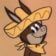I kinda sorta understand General Relativity with a mental spatial model.

I cannot understand Special Relativity that way - or any other way (although I've seen some good efforts). Telling me, "Here's the equation" is about as meaningful as saying that we do Standard Deviation by adding up the squares of each value's distance from the mean (followed by other operations).

Why square? Why not cube or to the 2.1 power or logarithm or square root? Near as I can tell, *it's arbitrary* (though there are technical advantages: easier math, works with Gaussian distributions, etc.)

The moral is: Don't be a slave to the equation.

An amusing thread questioning (and answering) why standard deviation involves squaring and then square-rooting can be found here:

1.Using the root-mean-square value for standard deviation is equivalent to using the Euclidean norm. There are other choices for norms (in other fields as well, not just statistics), but the Euclidean norm has several nice properties; in particular, it corresponds to our ordinary notions of "distance." So while it is certainly a choice to use the Euclidean norm, it's not an arbitrary choice.

1.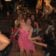Er, um, ... no.

1.I have five small cardboard boxes. Each one contains some cash, somewhere between \$1 and \$1000. Otherwise identical from the outside, each box is labeled with the amount of cash it contains. You may choose one box and take it home with you, no strings attached. Which one do you choose? If, like most people, you choose the box labeled \$1000, is that an arbitrary choice?

1.No, it's not to do with the Euclidean norm.

2.It is arbitrary. If we wanted to, we could take the sum absolute value of the differences from the mean (and some people -- for reasons I won't go into here -- argue that this is the better measure). The only requirement is that the differences are all non-negative, otherwise differences down could cancel differences up.

3.A Gaussian distribution occurs naturally when a measurement is made of a whole lot of small variables that add randomly. That is why Gaussian or normal distributions are so ubiquitous. A Gaussian distribution can be described by two variables, the centroid, and one describing the width. The root mean square standard deviation is convenient because when you add various Gaussian distributions together, the standard deviation of the resultant gaussian will be the root mean square of the standard deviation of the individual Gaussians that were combined. The whole root sum square thing comes out of all distributions of all the individual factors being independent (or orthogonal in mathematical sense), so that like adding perpendicular distances to get a total distance one uses the root sum square. I am an experimentalist physicist/engineer, so all you mathematicians please excuse my lack of rigor.

11.Then there’s the Feline Variation, in which the subject becomes infinitely more massive while at rest as time passes. Nothing is more massive than the Feline Variation until there is the introduction of the Rodent Component. Then, nothing moves as quickly as the Feline Variation, whatever its mass. The only math required is that, as the equation plays out, there must be 1 fewer rodent in the space controlled by the Feline Variation.

12.Remember, that even after all this is explained and worked out, a bipedal hominid (very few of them in fact) with a brain calibrated to escaping snakes and tigers and barely enough wherewithal to think into the future a few years thought all this out.

Now if we can survive the other proclivities of that brain, we might be able to utilize those thoughts.

13.Now do quantum mechanics in 700.

14.I bet you would never see a discussion like this in Tucker Carlson's blog, if he had one.

15.One nitpick, T is the stress energy tensor, not the metric tensor.

16.The idea that GR incorporates the notion of curvature in spacetime and that it is this curvature that is responsible for gravity is a common misconception.

What GR is, is a tensor theory. Now, you can interpret the field equations as curvature in the underlying spacetime, and that's certainly valid. But it is just as valid to interpret gravity as a tensor field, just as EM is a vector field.

Why does this matter? Because you can derive all of GR simply by positing a force-carrying particle of spin-2 in quantum field theory and then figuring out what that implies about the nature of the force its mediating; among other things, you get that it has to be a tensor field (by the same reasoning since the electromagnetic force is mediated by spin-1 particles, aka photons, it has to be a vector field.) You can even work out that spin-2 fields are always attractive while spin-1 fields may be either attractive or repulsive.

Good stuff. But not nearly as obvious or easy to visualize or 'intuitive' as the curved space explanation.

1.Picking nits here, but special relativity made EM a second order vector field. One of the very neat things about the Special theory is the way it replaces seperate vector E and B fields with a EM field tensor.

1.Well pick away! I'm just an algebraist who occasionally dabbled in low-topology.

17.Gravity might as well be magic. It's a force that... attracts. That's what it does, not what it is.

Even general relativity only describes it as mass traveling along warped space time.

1.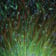Sheer charisma.

1.Sheer charisma.

We should rename it Trumpity. Or maybe MAGAvity.

18.It is all math, but it is useful to follow Einstein's thought processes before he arrived at (some of) the math. Einstein's inspirations were often thought experiments. What would happen if an observer looking at the clock on the tower in Zurich could chase the light rays reflected from the clock? As he moved faster and faster, the clock hands would appear to move slower and slower until, as he reached the speed of light, the hands would stop altogether. Voila - time dilatation!

After special relativity involving relative motion with constant velocity, Einstein wanted to go to generalized or accelerated motion. Special relativity showed that uniform motion (approaching the speed of light) led to contraction of length in the direction of motion. With generalized motion, he argued that space would bend (like a mollusc, in his words). Then he had what he said was the happiest thought of his life. He realized that his work on accelerated frames would carry over unchanged to gravitation which, after all, involves just another accelerated frame. And hence to the curved non-Euclidian geometry of general relativity.

19.Question: If Earth was denser, and time therefore slowed down from what it is now, would we age slower (assuming no untoward physiological effects from increased gravity)?

1.Time would pass more slowly for us from the point of view of an outside observer (e.g., a person on Mars), but we wouldn't notice anything different, because everything on Earth would slow down by the same amount. It's only when we travel to Mars, carrying our atomic clock with us, and compare our clock with theirs, that we discover the difference. (There are some other time-related effects caused by accelerating to high speed to make the trip, but that's a discussion for another time....)

As a real-world example of this, GPS relies on extremely accurate timekeeping. GPS satellites are a little farther from the center of the Earth than we are, so the force of gravity is slightly weaker, and their clocks do run at a different rate from ones on Earth. However, there are actually two relativistic effects in play for them: The reduced gravitational field causes the clocks to run slightly faster (from our point of view), about 45 microseconds per day (General Relativity). But the satellites are also orbiting at high speed, which makes their clocks run slightly slower (again from our point of view), about 7 microseconds per day (Special Relativity). The combined effect is that we perceive the clocks to run about 38 microseconds fast per day, and this discrepancy has to be taken into account for GPS to work properly.

2.Strictly speaking, it's not the gravity, it's the gravitational potential that causes time dilation. That's why time dilation can be so extreme even for a constant 1 g if you accelerate long (far) enough. You might want to check out Rindler coordinates for a nice picture of what's actually going on

20.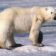Thank you Kevin. Very droll.

21.99 times out of 10* (sic) the expectation of “500 words or less” excludes formulas…

* this forum’s readers being among the 100th time out of 10.

22."...nothing can go faster than the speed of light..."

Except, apparently, all the alien ships currently visiting Earth.

23.Now do dark matter and dark energy. Number one son, the rocket scientist, jokes one is the stuff we dont understand that falls down and the other is the stuff we dont understand that falls up. Keep hoping for a better, concise explanation.

Steve

24.I'm sorry but just tossing out equations is a pretty far thing from "explaining" these theories. I've seen other writers explain, in reasonably intuitive ways, how these theories function in roughly the same amount of words but without relying on "here's the math, get it now, dummies?"

Does Mr. Drum need a new hobby or something, because this is a useless post.

25."it's all just math, baby."

Not quite true. It is also (extremely difficult and precise) physical experimentation that proves that the mathematical assumptions are correct.

26.I'd sure like someone to describe what a universe that isn't ruled by math would look like. Math was developed as a tool for thinking rigorously about things.